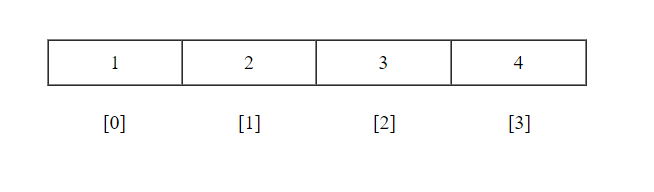# Sequences and Lists in Python

In Python, a sequence is a set of ordered list. They are differentiated by their index number. The index starts from zero. Two basic types of sequence in Python are list and tuple.## 1. Lists

• For creating new list use square brackets.
• In the list, it is not necessary that all list values should be of same Datatype (i.e, int, float, string,etc).
• Lists are mutable or we easily modify existing list.

Creating a list

For creating a list, just put values between the square bracket and separated by commas.

Syntax –

```[value1, value2, value3,...]
```
```list1 = [1,2,3,4]
list3 = ['h','e','l','l','0']
list4 = [4,"Jiten",'a',5]
```

Accessing list

For accessing values of the list, put index position inside square brackets.

Syntax –

```list-variablename[index]
```

For slicing,

```list-variablename[start-index : end-index]
```

start-index is from where to start selection, and end-index is index position where selection ends.

Example –

```list1 = [1,2,3,4]

print("list1 : ", list1)
print("list2[1:3] : ", list2[1:3])
print("list1[-2] : ",list1[-2])```

When we execute the above program, it will produce the following output –

```list1 : 3
list2[1:3] : ['Vishal', 'Vijay']
list1[-2] : 3```

Updating list

For updating list, select index position and assign value using assignment operator (=).

```list1 = [1,2,3,4]
list1 = 11
print(list1)
```

When we execute the above program, it will produce the following output –

```[1,2,11,4]
```

Deleting list item

In Python, we can use del statement for deleting existing list or delete list item by index.

Syntax –

`del list-name or del list-name[index]`

Example –

```list1 = [1,2,3,4]
del list1
print(list1)
```

Output

```[1,2,4]
```

List Functions

1. append – Adding a new value to existing list.
Syntax – `append(value)`

Example –

```list1 = [1,2,3,4]
list1.append(32)
print(list1)
```

Output

```[1,2,3,4,32]
```

2. count – Return the occurrence of value in existing list.
Syntax `count(value)`

Example

```list1 = [1,2,3,4,3,43]
c = list1.count(3)
print(c)
```

Output

`2`

3. clear – Clear the existing list values.
Syntax `clear()`

Example

```list1 = [43,3,22,11,54]
list1.clear()
print(list1)
```

Output

```[]
```

4. extend – Adding sequence to existing list.
Syntax `extend(sequence)`

Example

```list1 = [1,2,3,4]
list1.extend([5,6])
print(list1)
```

Output

`[1,2,3,4,5,6]`

5. insert – Insert value at given index.
Syntax `insert(index, value)`

Example

```list1 = [1,2,3,4]
list1.insert(2,54)
list1.insert(5,11)
print(list1)
```

Output

```[1,2,54,3,4,11]
```

6. index – Return the lowest index position of a value.
Syntax `index(value)`

Example

```list1 = [1,2,3,2,4]
a = list1.index(2)
print("position : ",a)
```

Output

```position : 1
```

7. pop – Remove the existing list value by index and return removing index value.
Syntax `pop(index)`

Example

```list1 = [1,2,3,4]
print(list1)
print(list1)
```

Output

```3
[1,2,4]
```

8. remove – Its also remove value from existing list by index, but it doesn’t return any value.
Syntax `remove(index)`

Example

```list1 = [55,21,3,13,7]
list1.remove(2)
print(list1)
```

Output

```[55,21,13,7]
```

9. reverse – Reverse the existing list.
Syntax `reverse()`

Example

```list1 = [1,2,3,4]
list1.reverse()
print(list1)
```

Output

```[4,3,2,1]
```

10. sort – Sort the existing list.
Syntax `sort()`

Example

```list1 = [43,3,22,11,54]
list1.sort()
print(list1)
```

Output

```[3, 11, 22, 43, 54]
```

Some others Functions

1. len – Return the total length of a list.

Syntax `len(list)`

Example

```list1 = [43,3,22,11,54]
print("Length : ",len(list1))
```

Output

`Length : 5`

2. max – Return the max value from a list.

Syntax `max(list)`

Example

```list1 = [43,3,22,11,54]
print("Max : ",max(list1))
```

Output

`Max : 54`

3. min – Return the min value from a list.

Syntax `min(list)`

Example

```list1 = [43,3,22,11,54]
print("Min : ",min(list1))
```

Output

`Min : 3`

4. list – Convert a tuple into a list.

Syntax `list(seq)`

Example

```tuple1 = (43,3,22,11,54)
list1 = list(tuple1)
print("tuple1 : ",tuple1)
print("list1 : ",list1)```

Output

```tuple1 : (43,3,22,11,54)
list1 : [43,3,22,11,54]```

## 2. Tuple

• For creating a new tuple use parentheses.
• In tuple, it is not necessary that all values should be of same datatype (i.e, int, float, string,etc).
• Tuples are immutable or we can’t modify values of a tuple.

Creating a list

For creating a tuple, just put values between parentheses and separated by commas.

Syntax –

```(value1,value2,value3,...)
```

### Accessing a tuple

For accessing values of a tuple, put index position inside square brackets.

Syntax –

```tuple-variablename[index]
```

For slicing,

```tuple-variablename[start-index : end-index]
```

start-index is from where to start selection, and end-index is index position where selection ends.

Example –

```tuple1 = [22,1,34,54]

print("tuple1 : ", tuple1)
print("tuple2[1:3] : ", tuple2[1:3])
print("tuple1[-2] : ",tuple1[-2])```

When we execute the above program, it will produce the following output –

```tuple1 : 3
tuple2[1:3] : ['Vishal', 'Vijay']
tuple1[-2] : 34```

Tuple Functions

1. count – Return the occurrence of value in existing tuple.
Syntax`count(value)`

Example

```tuple1 = [12,1,54,41,1]
print("count : ",tuple1.count(1))
```

Output

`count : 2`

2. index – Return the lowest index position of a value.
Syntax`index(value)`

Example

```tuple1 = [12,1,54,41,1]
print("position : ",tuple1.index(1))
```

Output

`count : 2`

Some others Functions

Syntax `len(tuple)`

Example

```tuple1 = (43,3,22,11,54)
print("Length : ",len(tuple1))
```

Output

`Length : 5`

2. max – Return max value from tuple.

Syntax `max(tuple)`

Example

```tuple1 = [43,3,22,11,54]
print("Max : ",max(tuple1))
```

Output

`Max : 54`

3. min – Return min value from tuple.

Syntax `min(tuple)`

Example

```tuple1 = [43,3,22,11,54]
print("Min : ",min(tuple1))
```

Output

`Min : 3`

4. tuple – Convert a list into a tuple.

Syntax `tuple(seq)`

Example

```list1 = [43,3,22,11,54]
tuple1 = tuple(list1)
print("list1 : ",list1)
print("tuple1 : ",tuple1)```

Output

```list1 : [43,3,22,11,54]
tuple1 : (43,3,22,11,54)```